# 论文阅读 - Cascade R-CNN: Delving into High Quality Object Detection

Github - open-mmlab/mmdetection(PyTorch)

Github - tensorpack/tensorpack(TensorFlow)

 - 由于正样本的指数级衰减，导致训练的过拟合(正样本被过滤，导致样本数量过少).

 - 由于训练时采用的 IoU 和检测的最优 IoU 的不一致性，导致 mismatch.

Cascade R-CNN 方法是采用逐渐递增的 IoU 阈值训练的序列的检测器，其序列化地选择更优的正样本和负样本. 其训练是 stage-by-stage 的.

## 1. 问题描述

 - 目标检测器需要解决类别识别问题，以从背景中区分前景目标，并分配给目标物体以正确的类别标签.

 - 目标检测器需要解决物体定位问题，以分配给不同目标物体以精确的边界框.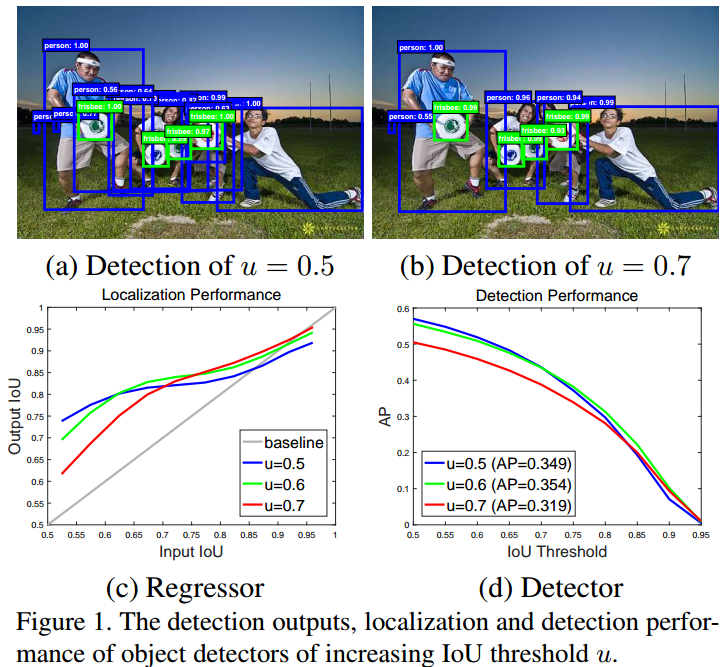Cascade R-CNN 的基本思想是，单一检测器只能是对单一 IoU 层次是最优的. Cascade R-CNN 考虑了对给定 IoU 阈值的优化. 即，采用级联的方式，首先利用 IoU=0.5 的网络，提升输入的 proposals；比如 IoU 提升到 0.6，再利用IoU=0.6的网络进一步的提升；比如 IoU 提升到 0.7，再利用IoU=0.7 的网络进行提升. 逐步的提升检测器的精度.

Cascade R-CNN is a multi-stage extension of the R-CNN, where detector stages deeper into the cascade are sequentially more selective against close false positives. The cascade of R-CNN stages are trained sequentially, using the output of one stage to train the next. This is motivated by the observation that the output IoU of a regressor is almost invariably better than the input IoU.

## 2. 目标检测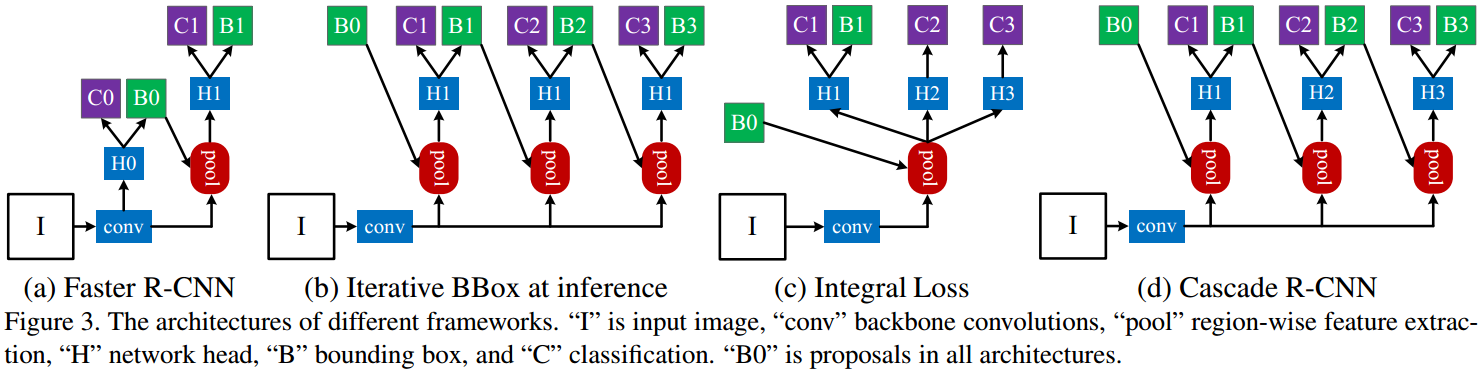- first stage，H0 为 proposal sub-network，用于对整个输入图像进行处理，输出初步的检测结果，即：object proposals. 如 RPN.

 - second stage，H2 为 RoI 检测 sub-network，用于对 object proposals 进行处理，记为：detection head.

 - final stage，C 为 classification score；B 为 bounding box，分别对应到每个 RoI 的输出.

### 2.1. 边界框回归

$$\mathcal{R}_{loc}[f] = \sum_{i=1}^N L_{loc}(f(x_i, \mathbf{b}_i), \mathbf{g}_i)$$

$$\begin{cases} \delta_x = \frac{g_x - b_x}{b_w} \\ \delta_y = \frac{g_y - b_y}{b_h} \\ \delta_w = log(\frac{g_w}{b_w}) \\ \delta_h = log(\frac{g_h}{b_h}) \end{cases}$$

$$\begin{cases} \delta_x' = \frac{\delta_x - \mu_x}{\sigma_x} \\ \delta_y' = \frac{\delta_y - \mu_y}{\sigma_y} \\ \delta_w' = \frac{\delta_w - \mu_w}{\sigma_w} \\ \delta_h' = \frac{\delta_h - \mu_h}{\sigma_h} \end{cases}$$

### 2.2. 迭代边界框回归

$$f'(x, \mathbf{b}) = f \circ f \circ ... \circ f(x, \mathbf{b})$$

 - 如图 Figure1 中所示，采用 IoU=0.5 训练的回归器 $f$ ，其对于 IoU 更高的图像检测效果是非最优的. 实际上，对于 IoU 大于 0.85 的情况，其反而会对边界框有负影响.

 - 如下图 Figure2 所示，每次迭代后边界框的分布变化比较大. 尽管对于初始分布而言，回归器是最优的，但后面迭代后的可能非最优.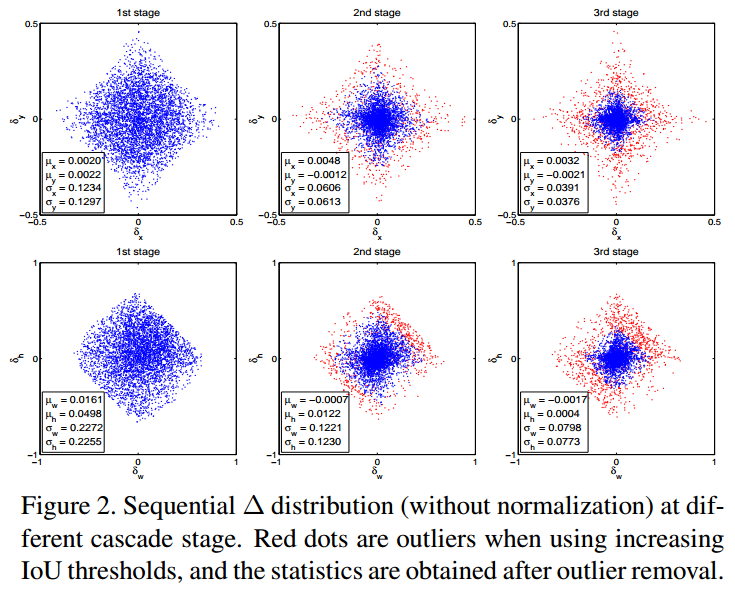### 2.3. 边界框分类

$h(x)$ 是 M+1 维的关于类别的后验分布估计：

$$h_k(x) = p(y=k|x)$$

$$\mathcal{R}_{cls}[h] = \sum_{i=1}^N L_{cls}(h(x_i), y_i)$$

### 2.4. 检测质量

$$x = \begin{cases} g_y &\text{ } IoU(x, g) \geq u \\ 0 &\text{otherwise } \end{cases}$$

$$L_{cls}(h(x), y) = \sum_{u \in U} L_{cls}(h_u(x), y_u)$$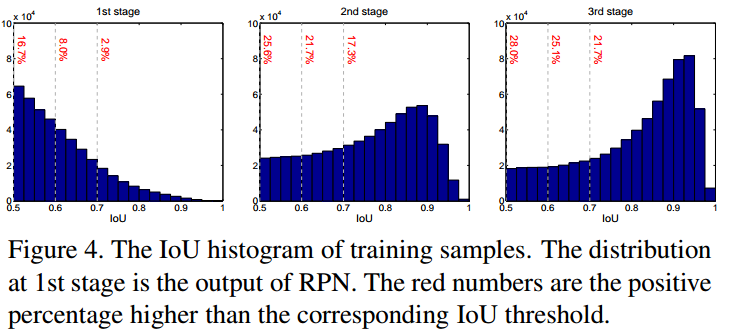$$f(x, \mathbf{b}) = f_T \circ f_{T-1} \circ ... \circ f_1(x, \mathbf{b})$$

 - iterative BBox 是用于提升边界框的后处理流程，而 Cascade R-CNN的回归是一种重采样流程，其会改变不同 stages 处理后的 proposals 的分布，训练时不同 stage 的输入数据分布是不同的.

 - 回归器 $\lbrace f_T, f_{T-1}, ..., f_1 \rbrace$ 是对于不同 stages 的重采样分布(resampled distributions) 进行优化的.

$$L(x^t, g) = L_{cls}(h_t(x^t), y^t) + \lambda[y^t \geq 1]L_{loc}(f_t(x^t, \mathbf{b}^t), \mathbf{g})$$

## 4. Results

### 4.1. 实现细节

Cascade R-CNn 包含 4 个 stages，第一个 stage 用于 RPN，其它三个 stages 采用的 IoU 阈值为 $U = \lbrace 0.5, 0.6, 0.7 \rbrace$.

### 4.2. 论文结果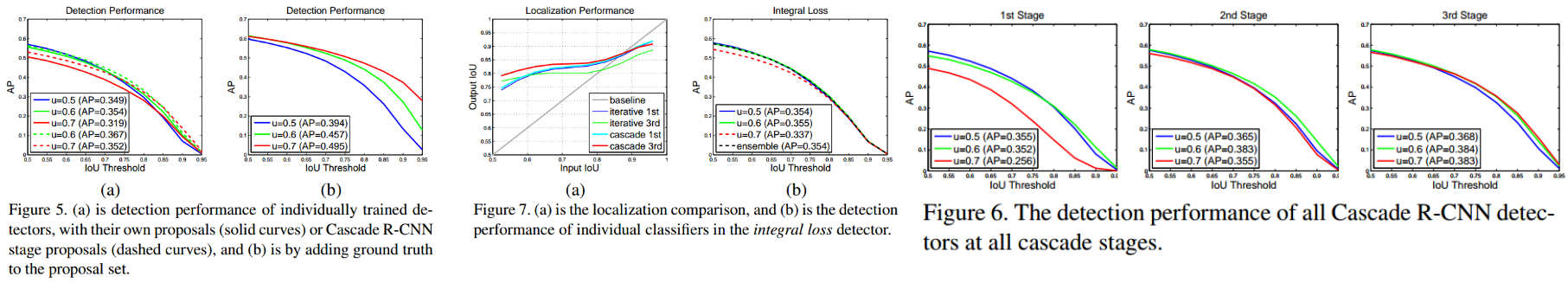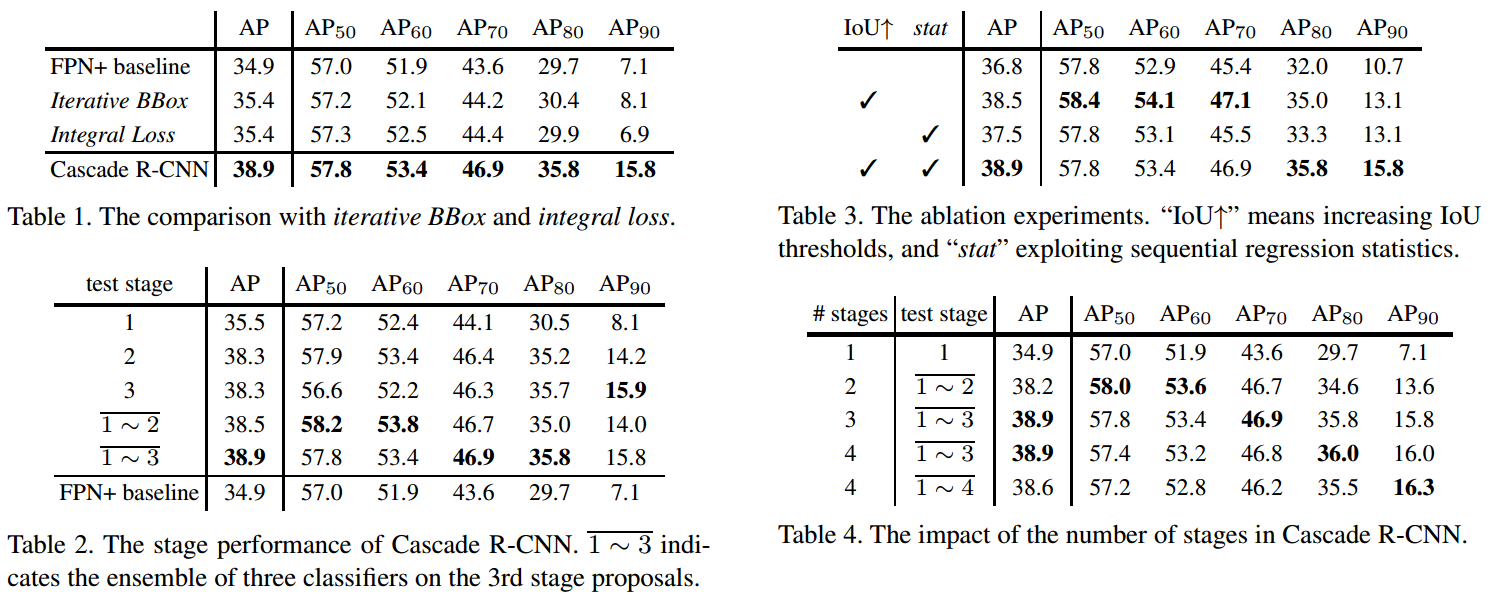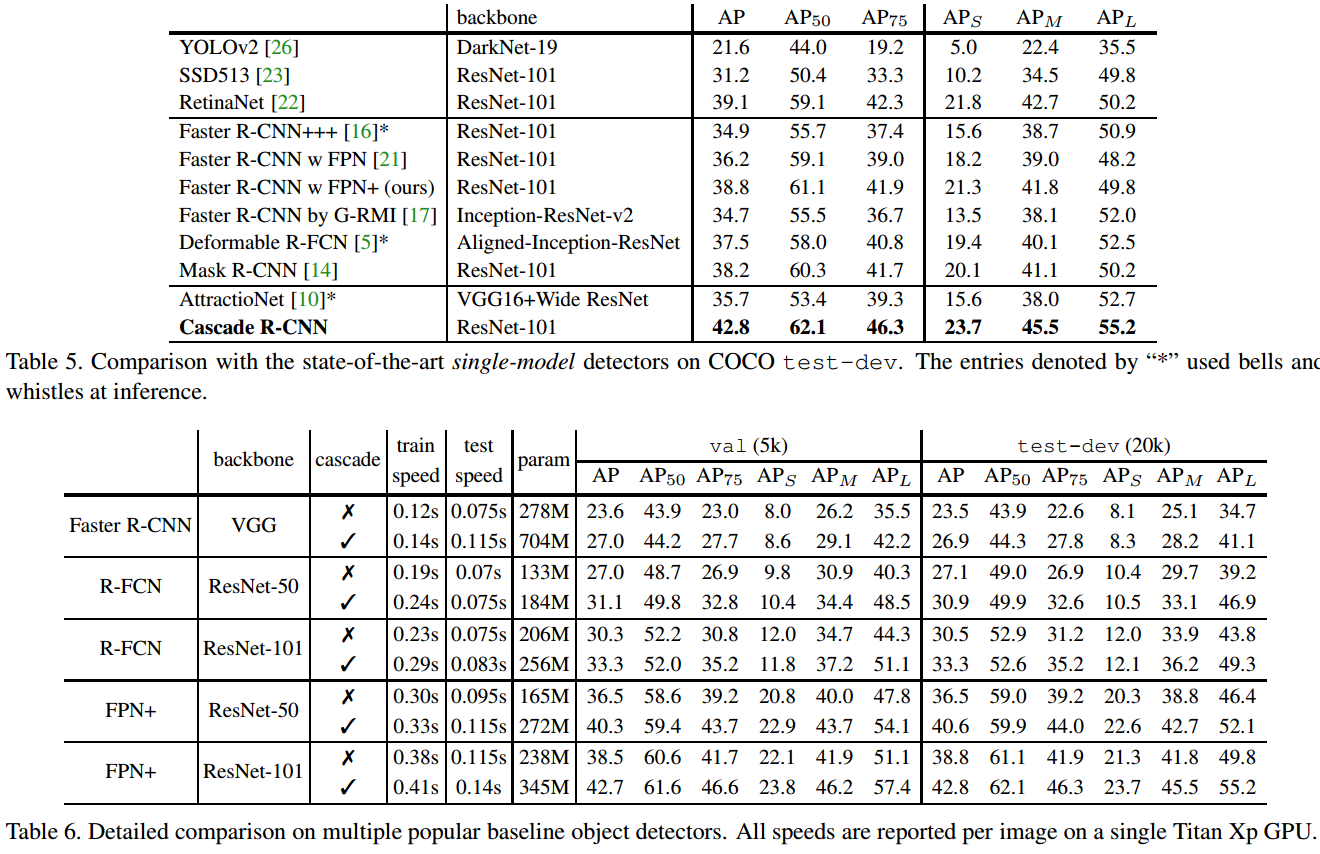## 5. 相关

Last modification：July 30th, 2019 at 02:40 pm